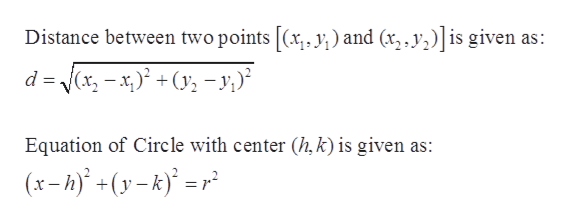# Find the equation of the circle with center at (−2, 0) and that passes through the point whose coordinates are (−4, −5).

Question
6 views

Find the equation of the circle with center at (−2, 0) and that passes through the point whose coordinates are (−4, −5).

check_circle

Step 1

Given:

Center of the circle is (–2, 0).

Point through which the circle is passing: (–4, –5)

Step 2

Formula and concept used:help_outlineImage TranscriptioncloseDistance between two points [(x, y; ) and (x, , y,)|is given as: d = (x, - x,)° +(y; - y;)? Equation of Circle with center (h,k) is given as: (x- h)' +(y-k) =r² fullscreen
Step 3

The given two points in th...

### Want to see the full answer?

See Solution

#### Want to see this answer and more?

Solutions are written by subject experts who are available 24/7. Questions are typically answered within 1 hour.*

See Solution
*Response times may vary by subject and question.
Tagged in
MathAlgebra

### Other